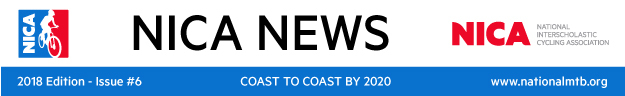# President's Note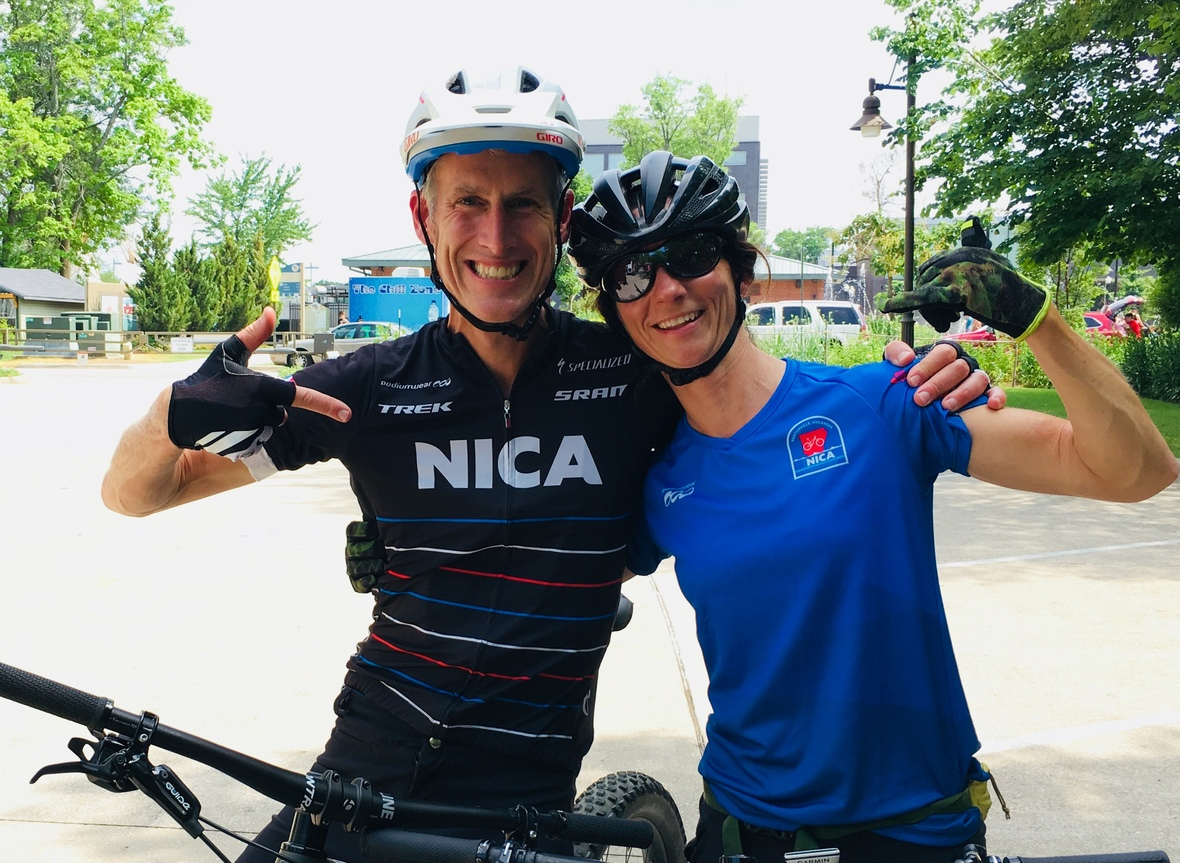Getting ready to ride with West Virginia League Director, Cassie Smith, in Bentonville, Arkansas at the 2018 NICA National Conference.

If there’s one word I can use to describe the sentiment after participating in NICA’s 8th Annual National Conference in Arkansas in late June, it is definitely “Wow!” This year’s conference was our largest yet, with over 150 attendees including league leadership, coach supporters and event production staff, and after having spent five days in Bentonville, Arkansas with this group of enthusiastic individuals, I am excited about the current and future state of NICA across the United States. Due to our gracious host, the Arkansas Interscholastic Cycling League and Visit Bentonville, participants had the opportunity to enjoy some fantastic rides on trails starting from downtown Bentonville. Likewise, through the leadership of Kyla Templeton, Arkansas League Director, and her team of parent volunteers, conference attendees were able to meet and mingle with local Arkansas League students and coaches. It is easy to see that this is a community that has truly embraced the sport of mountain biking and integrated it into the fabric of their everyday lives.

As you’ll read below, at this year’s conference, thanks to support from the Walton Family Foundation, we launched a new program, Girls Riding Together (GRiT), aimed to get #moregirlsonbikes. Also, again through support from the Walton Family Foundation and REI, we are re-energizing our Teen Trail Corps trail stewardship program. Amazing guest speakers, such as John Burke, President of Trek Bicycles, helped energize and inspire NICA leadership to grow the sport of youth mountain biking. Mr. Burke also announced Trek’s strengthened partnership with NICA to support creation of new NICA leagues, bolster the efforts of current leagues and enhance our coach licensing and training programs.

This month, we are also recognizing our 2017 NICA National Award recipients and welcoming THREE new states to the NICA community. The future of NICA is bright, and I hope you are just as excited as I am about the outlook of interscholastic mountain biking across the United States!

With Gratitude,
Austin

 table div table+table+table+table div table{width:100%;padding:0}table div table+table+table+table div table img{width:96.23%;padding:0;float:none}table div table+table+table+table div table td{width:100%;padding:0 1.88% 18px}/* styles */# Welcome Montana, Florida and Indiana!

We are excited to announce NICA's three newest emergent leagues: Montana, Florida and Indiana! Welcome to the NICA community! These new leagues bring with them extensive youth development experience and talented individuals. The are now 25 NICA leagues across the country - read more about these new leagues HERE.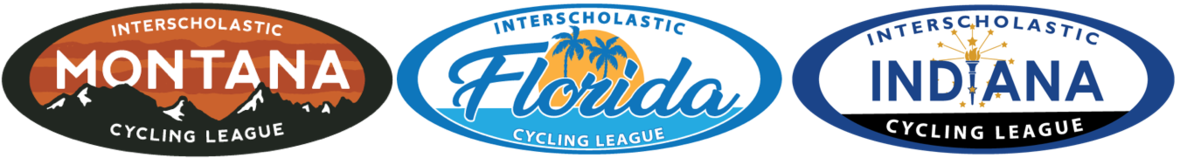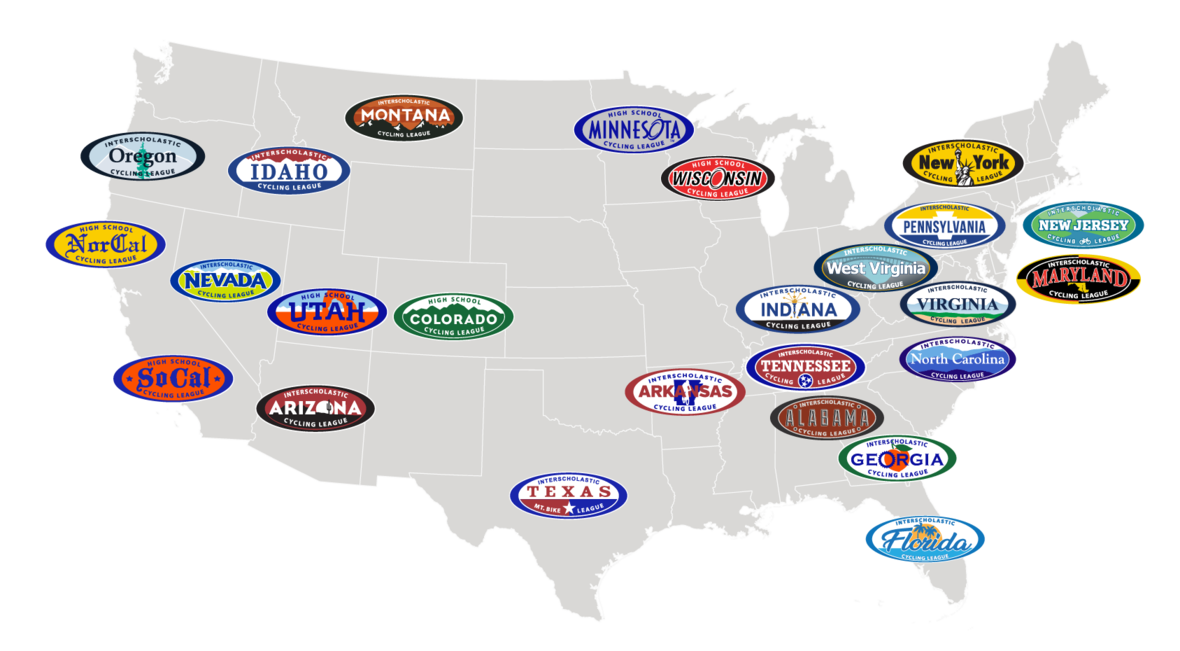table div table+table+table+table+table+table+table+table div table{width:100%;padding:0}table div table+table+table+table+table+table+table+table div table img{width:96.23%;padding:0;float:none}table div table+table+table+table+table+table+table+table div table td{width:100%;padding:0 1.88% 18px}/* styles */# What's Happening Across America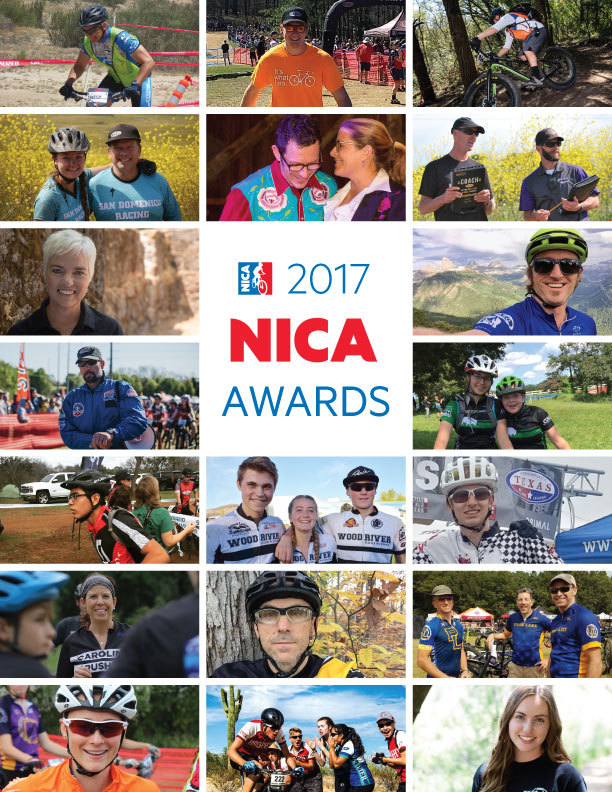## NICA Award Recipients Announced

Congratulations to our NICA Award recipients for 2017! The NICA Awards were established in 2010 to honor student-athletes, coaches, volunteers and supporters who have made outstanding contributions toward furthering the interscholastic mountain biking movement across the country. Meet our 2017 recipients HERE.

## 2018 National Conference a Success!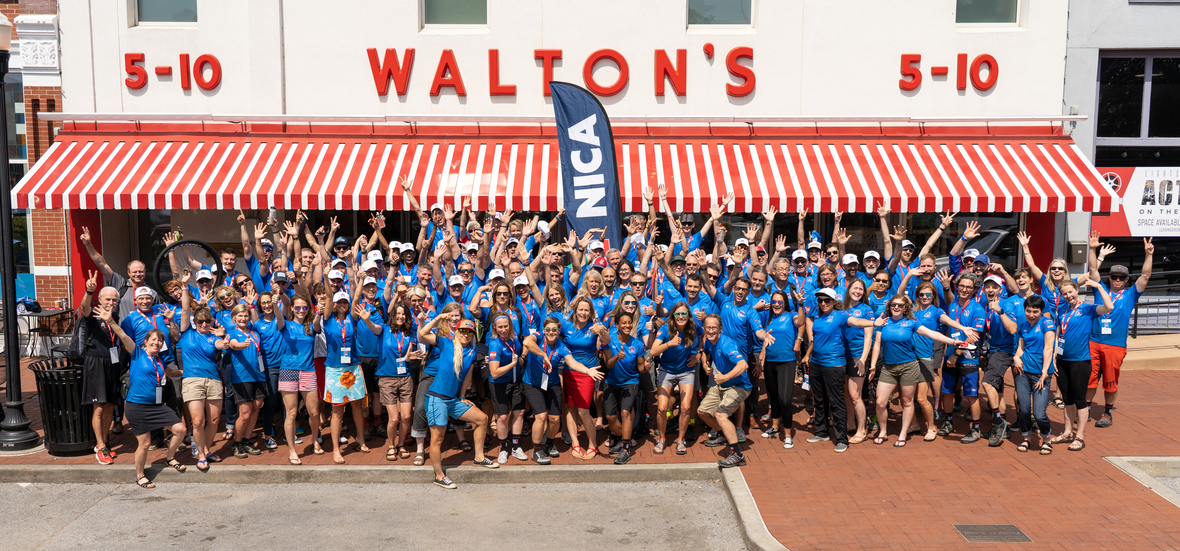2018 National Conference Attendees. Photo: Todd Bauer / TMB Images
 /* styles */ Last month, NICA hosted its annual conference in Bentonville, Arkansas. This year’s event brought over 150 leaders together from 22 states and regions to strategize, connect and continue to expand NICA’s efforts to promote youth development through mountain biking across the United States. You can read more about the 2018 conference HERE.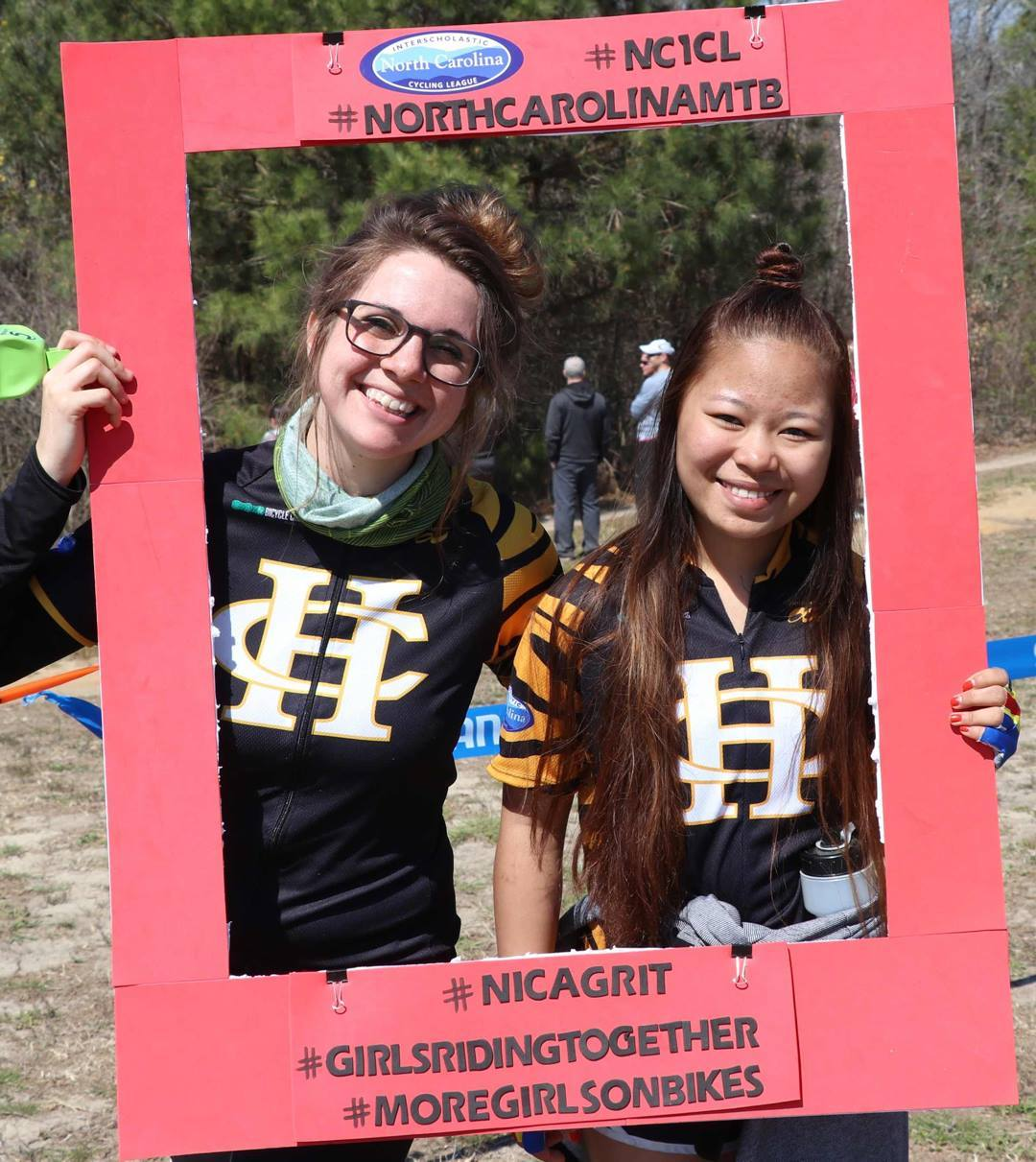Photo: Deborah Hage

## Girls Riding Together (GRiT) Program Launches

One of the highlights of the National Conference was the launch of NICA's Girls Riding Together (GRiT) program. NICA GRiT is aimed at increasing female participation in interscholastic mountain biking by 10% over the next five years. Learn more about NICA GRiT HERE.## NICA receives support from Trek Bicyles and Walton Family Foundation

NICA Leagues are now receiving direct support from Trek Bicycles and NICA programs have been bolstered by a generous grant from the Walton Family Foundation. Learn more about their support HERE.

 table div table+table+table+table+table+table+table+table+table+table+table+table+table+table+table+table div table{width:100%;padding:0}table div table+table+table+table+table+table+table+table+table+table+table+table+table+table+table+table div table img{width:96.23%;padding:0;float:none}table div table+table+table+table+table+table+table+table+table+table+table+table+table+table+table+table div table td{width:100%;padding:0 1.88% 18px}/* styles */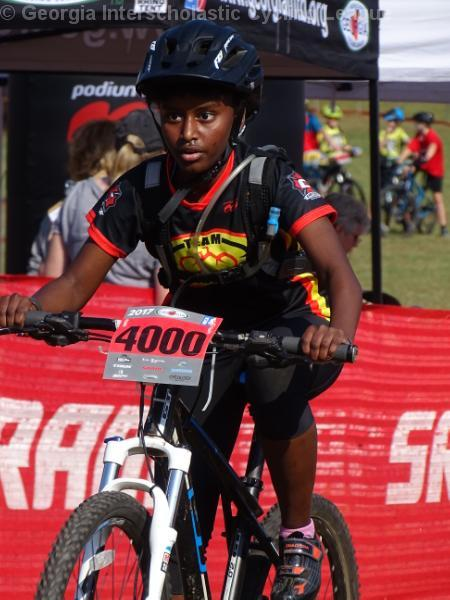# Meet Louisa - GRiT Ambassador

With the official launch of NICA's Girls Riding Together (GRiT) program - we wanted to catch up with one of our student-athletes who has been involved with GRiT program activities in her local league!

Meet Louisa - a 6th grader with the Georgia Interscholastic Cycling League. Louisa rides for the Gilmer Cartecay Composite Team (aka Team Cartecay) and is a GRiT Ambassador for the Georgia League this season!

We caught up with Louisa HERE to learn about her experience with NICA GRiT and why she's excited about #moregirlsonbikes!

 table div table+table+table+table+table+table+table+table+table+table+table+table+table+table+table+table+table+table div table{width:100%;padding:0}table div table+table+table+table+table+table+table+table+table+table+table+table+table+table+table+table+table+table div table img{width:96.23%;padding:0;float:none}table div table+table+table+table+table+table+table+table+table+table+table+table+table+table+table+table+table+table div table td{width:100%;padding:0 1.88% 18px}/* styles */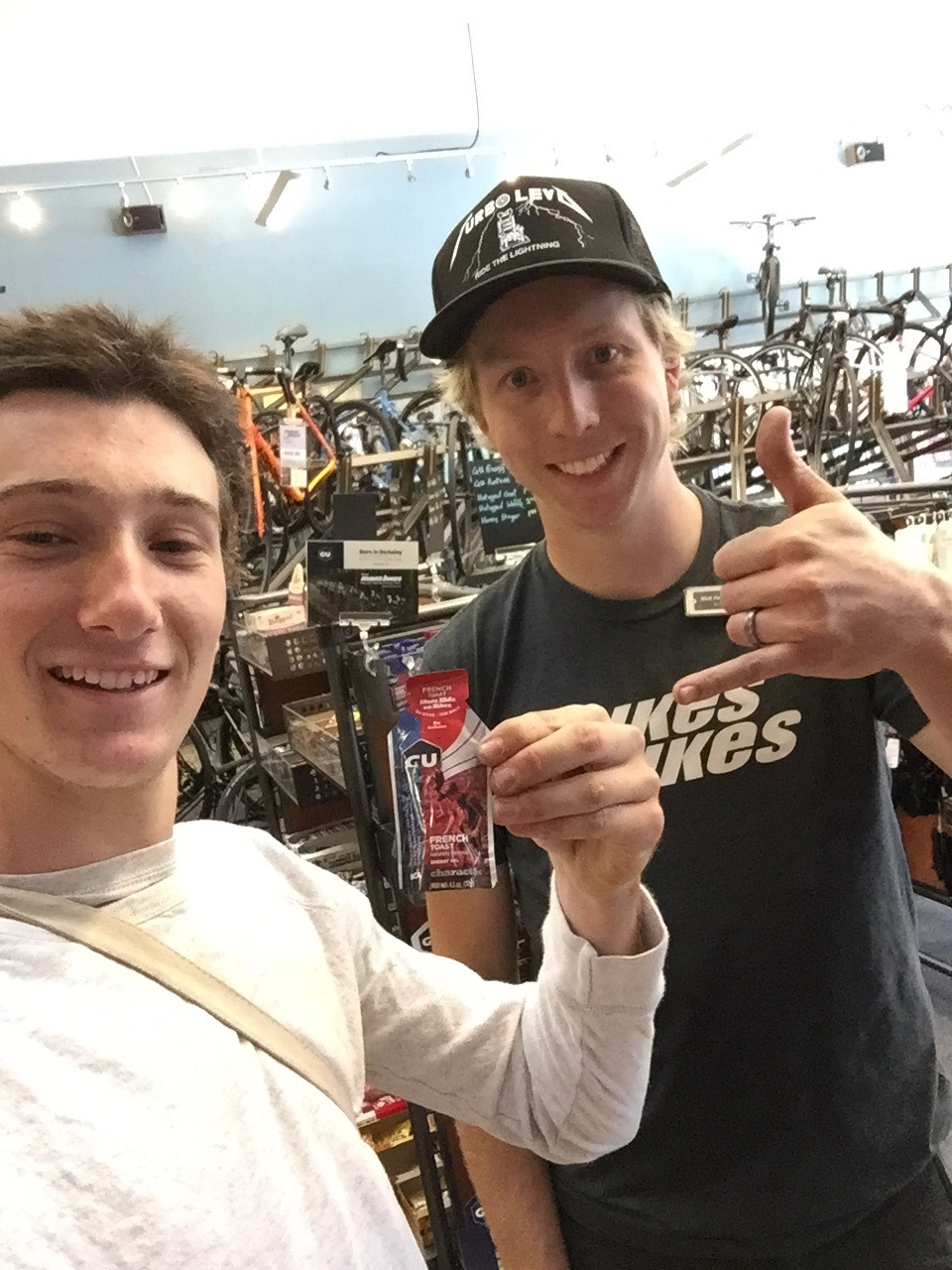Find French Toast at your local bike shop!

# Have you tried FRENCH TOAST Yet?

This past spring, GU Energy Labs worked with NICA Student-Athletes to create a new flavor of energy gel! 10% of all proceeds will go to support NICA and our mission to put #morekidsonbikes! Visit your local shop to try it out today if you haven't already! Learn more and watch the cool video HERE. #GUFORIT!

 table div table+table+table+table+table+table+table+table+table+table+table+table+table+table+table+table+table+table+table+table div table{width:100%;padding:0}table div table+table+table+table+table+table+table+table+table+table+table+table+table+table+table+table+table+table+table+table div table img{width:96.23%;padding:0;float:none}table div table+table+table+table+table+table+table+table+table+table+table+table+table+table+table+table+table+table+table+table div table td{width:100%;padding:0 1.88% 18px}/* styles */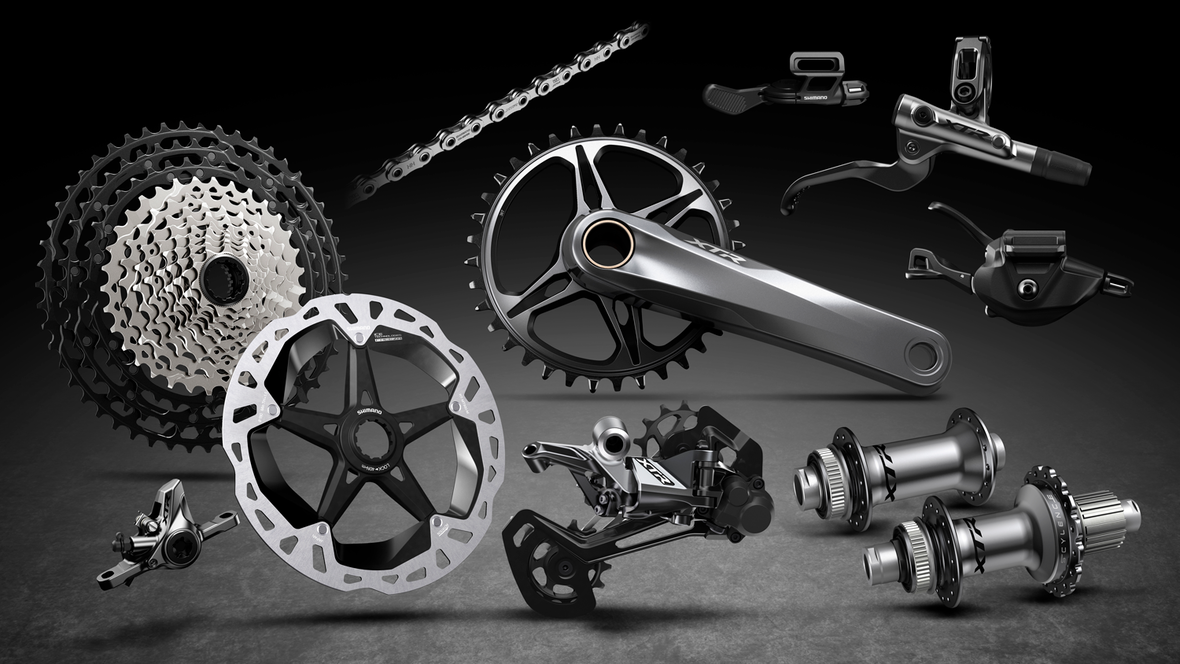# Introducing Shimano XTR M9100

Need a new drivetrain this fall? Look no further. Building on their legacy of innovation, Shimano is proud to debut their new 12-speed ultra-efficient drivetrain featuring a 10-51t cassette while offering superior shifting and braking systems specifically tuned to meet the demands of all racers. Check it out today at http://ridextr.com/!

 table div table+table+table+table+table+table+table+table+table+table+table+table+table+table+table+table+table+table+table+table+table+table div table{width:100%;padding:0}table div table+table+table+table+table+table+table+table+table+table+table+table+table+table+table+table+table+table+table+table+table+table div table img{width:96.23%;padding:0;float:none}table div table+table+table+table+table+table+table+table+table+table+table+table+table+table+table+table+table+table+table+table+table+table div table td{width:100%;padding:0 1.88% 18px}/* styles */# NICA Athlete Discount at Oz Trails Off-Road

Ride the Inaugural Oz Trails Off-Road in Bentonville, Arkansas this October 5 - 7 (Columbus Day Weekend)!

Featuring 3 distance options to choose from (25, 35 or 50 miles) and packed with popular Northwest Arkansas trails including The Back 40 Loop, All American Trail, SBAT, and Flo Ride - you don't want to miss this opportunity to ride what some are calling the new Mountain Bike Mecca of America.

A longtime supporter of NICA, Epic Rides proudly offers \$60 off for NICA Student-Athletes & Juniors and 50% off Team Support Booth Space for NICA teams in the Bike & Gear Expo.

For Registration & Complete event information visit epicrides.com

 table div table+table+table+table+table+table+table+table+table+table+table+table+table+table+table+table+table+table+table+table+table+table+table+table div table{width:100%;padding:0}table div table+table+table+table+table+table+table+table+table+table+table+table+table+table+table+table+table+table+table+table+table+table+table+table div table img{width:96.23%;padding:0;float:none}table div table+table+table+table+table+table+table+table+table+table+table+table+table+table+table+table+table+table+table+table+table+table+table+table div table td{width:100%;padding:0 1.88% 18px}/* styles */# Planet Bike Support for NICA GRiT

Planet Bike is are proud to support the mission of NICA’s GRiT program that inspires young women to build confidence, strength of character, and empowerment through off-road cycling. They love the balance the bicycle brings to the world and its power to make people, communities and the planet healthier. In addition to creating products that make cycling safer and more enjoyable, Planet Bike pledges, time, resources and profits to organizations working for sustainable transportation solutions.

NICA Student-Athletes - check in with your coaches about receiving a special discount from Planet Bike!

 table div table+table+table+table+table+table+table+table+table+table+table+table+table+table+table+table+table+table+table+table+table+table+table+table+table+table div table{width:100%;padding:0}table div table+table+table+table+table+table+table+table+table+table+table+table+table+table+table+table+table+table+table+table+table+table+table+table+table+table div table img{width:96.23%;padding:0;float:none}table div table+table+table+table+table+table+table+table+table+table+table+table+table+table+table+table+table+table+table+table+table+table+table+table+table+table div table td{width:100%;padding:0 1.88% 18px}/* styles */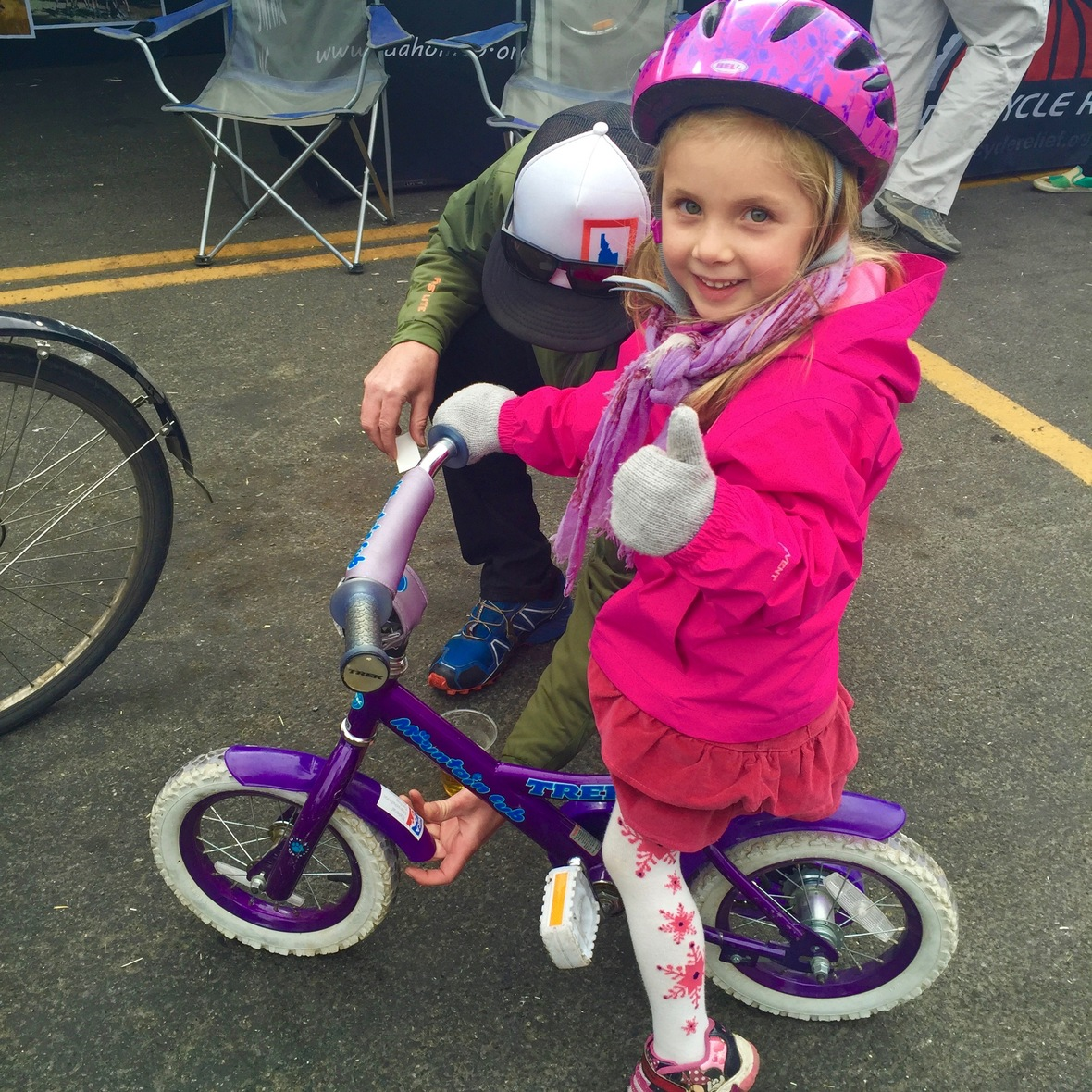Ready for a new set of wheels? Get in-store credit for your old bike at participating Trek Bicycle Retail stores! Bring in your old bike and receive Bicycle Blue Book value as in-store credit toward the purchase of a new ride. Details HERE.

 table div table+table+table+table+table+table+table+table+table+table+table+table+table+table+table+table+table+table+table+table+table+table+table+table+table+table+table+table div table{width:100%;padding:0}table div table+table+table+table+table+table+table+table+table+table+table+table+table+table+table+table+table+table+table+table+table+table+table+table+table+table+table+table div table img{width:96.23%;padding:0;float:none}table div table+table+table+table+table+table+table+table+table+table+table+table+table+table+table+table+table+table+table+table+table+table+table+table+table+table+table+table div table td{width:100%;padding:0 1.88% 18px}/* styles */# NICA is Hiring!

We're looking for a few talented and driven individuals who also happen to be stoked on youth development through mountain biking to join our growing team! We have have openings in our accounting, programs and development departments! Check out our openings HERE and apply today!

 table div table+table+table+table+table+table+table+table+table+table+table+table+table+table+table+table+table+table+table+table+table+table+table+table+table+table+table+table+table+table div table{width:100%;padding:0}table div table+table+table+table+table+table+table+table+table+table+table+table+table+table+table+table+table+table+table+table+table+table+table+table+table+table+table+table+table+table div table img{width:96.23%;padding:0;float:none}table div table+table+table+table+table+table+table+table+table+table+table+table+table+table+table+table+table+table+table+table+table+table+table+table+table+table+table+table+table+table div table td{width:100%;padding:0 1.88% 18px}/* styles */## We LOVE seeing what our student-athletes, teams, coaches and leagues are up to across the country. Here are some of the highlights from the past month!

Do you have a great photo worthy of inclusion in a future issue of NICA News? If so, please email to media@nationalmtb.org and you may see it published! And don't forget - when you're posting your photos to tag @nationalmtb #morekidsonbikes for a chance to see your photo in NICA News!

 table.module-31{width:75.66%;padding:0}table div table+table+table+table+table+table+table+table+table+table+table+table+table+table+table+table+table+table+table+table+table+table+table+table+table+table+table+table+table+table+table+table div table{width:75.66%;float:none;margin-left:auto;margin-right:auto;padding:0}table div table+table+table+table+table+table+table+table+table+table+table+table+table+table+table+table+table+table+table+table+table+table+table+table+table+table+table+table+table+table+table+table div table a{border:0 none;text-decoration:none}table div table+table+table+table+table+table+table+table+table+table+table+table+table+table+table+table+table+table+table+table+table+table+table+table+table+table+table+table+table+table+table+table div table img{width:100%!important;border:0 none;text-decoration:none}table div table+table+table+table+table+table+table+table+table+table+table+table+table+table+table+table+table+table+table+table+table+table+table+table+table+table+table+table+table+table+table+table div table td{width:100%;padding:0}/* styles */
 /* styles */ @southdavismtbteam rider @alyss.rich shows how much fun switchbacks are at the Wild Rose Trails. When you climb about 300 feet in a 1.7 mile loop you're going to have a fun ride down!
 table.module-33{width:75.47%;padding:0}table div table+table+table+table+table+table+table+table+table+table+table+table+table+table+table+table+table+table+table+table+table+table+table+table+table+table+table+table+table+table+table+table+table+table div table{width:75.47%;float:none;margin-left:auto;margin-right:auto;padding:0}table div table+table+table+table+table+table+table+table+table+table+table+table+table+table+table+table+table+table+table+table+table+table+table+table+table+table+table+table+table+table+table+table+table+table div table a{border:0 none;text-decoration:none}table div table+table+table+table+table+table+table+table+table+table+table+table+table+table+table+table+table+table+table+table+table+table+table+table+table+table+table+table+table+table+table+table+table+table div table img{width:100%!important;border:0 none;text-decoration:none}table div table+table+table+table+table+table+table+table+table+table+table+table+table+table+table+table+table+table+table+table+table+table+table+table+table+table+table+table+table+table+table+table+table+table div table td{width:100%;padding:0}/* styles */
 /* styles */ @georgiamtbleague riders having fun at a NICA GRiT event!
 table.module-35{width:75.47%;padding:0}table div table+table+table+table+table+table+table+table+table+table+table+table+table+table+table+table+table+table+table+table+table+table+table+table+table+table+table+table+table+table+table+table+table+table+table+table div table{width:75.47%;float:none;margin-left:auto;margin-right:auto;padding:0}table div table+table+table+table+table+table+table+table+table+table+table+table+table+table+table+table+table+table+table+table+table+table+table+table+table+table+table+table+table+table+table+table+table+table+table+table div table a{border:0 none;text-decoration:none}table div table+table+table+table+table+table+table+table+table+table+table+table+table+table+table+table+table+table+table+table+table+table+table+table+table+table+table+table+table+table+table+table+table+table+table+table div table img{width:100%!important;border:0 none;text-decoration:none}table div table+table+table+table+table+table+table+table+table+table+table+table+table+table+table+table+table+table+table+table+table+table+table+table+table+table+table+table+table+table+table+table+table+table+table+table div table td{width:100%;padding:0}/* styles */
 /* styles */ @simicompositemtbteam all smiles at the recent @socalleague Mammoth Summer Camp!
 table.module-37{width:75.66%;padding:0}table div table+table+table+table+table+table+table+table+table+table+table+table+table+table+table+table+table+table+table+table+table+table+table+table+table+table+table+table+table+table+table+table+table+table+table+table+table+table div table{width:75.66%;float:none;margin-left:auto;margin-right:auto;padding:0}table div table+table+table+table+table+table+table+table+table+table+table+table+table+table+table+table+table+table+table+table+table+table+table+table+table+table+table+table+table+table+table+table+table+table+table+table+table+table div table a{border:0 none;text-decoration:none}table div table+table+table+table+table+table+table+table+table+table+table+table+table+table+table+table+table+table+table+table+table+table+table+table+table+table+table+table+table+table+table+table+table+table+table+table+table+table div table img{width:100%!important;border:0 none;text-decoration:none}table div table+table+table+table+table+table+table+table+table+table+table+table+table+table+table+table+table+table+table+table+table+table+table+table+table+table+table+table+table+table+table+table+table+table+table+table+table+table div table td{width:100%;padding:0}/* styles */
 /* styles */ So much fun for @norcalleague Coach @fletcher_morgan and this crew of NICA legends at the 2018 NICA National Conference!
 table div table+table+table+table+table+table+table+table+table+table+table+table+table+table+table+table+table+table+table+table+table+table+table+table+table+table+table+table+table+table+table+table+table+table+table+table+table+table+table+table div table{width:100%;padding:0}table div table+table+table+table+table+table+table+table+table+table+table+table+table+table+table+table+table+table+table+table+table+table+table+table+table+table+table+table+table+table+table+table+table+table+table+table+table+table+table+table div table img{width:96.23%;padding:0;float:none}table div table+table+table+table+table+table+table+table+table+table+table+table+table+table+table+table+table+table+table+table+table+table+table+table+table+table+table+table+table+table+table+table+table+table+table+table+table+table+table+table div table td{width:100%;padding:0 1.88% 18px}/* styles */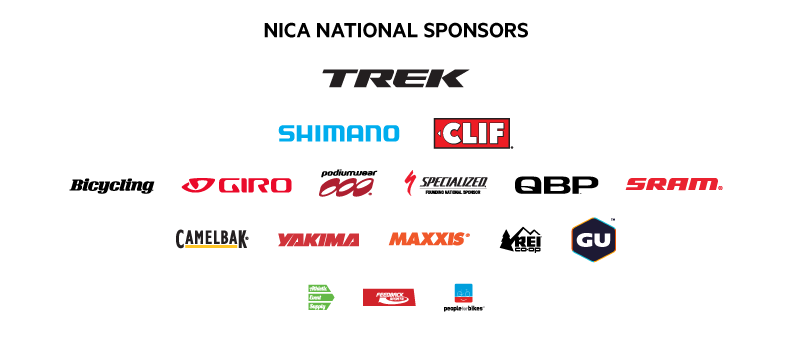Like   Tweet   in